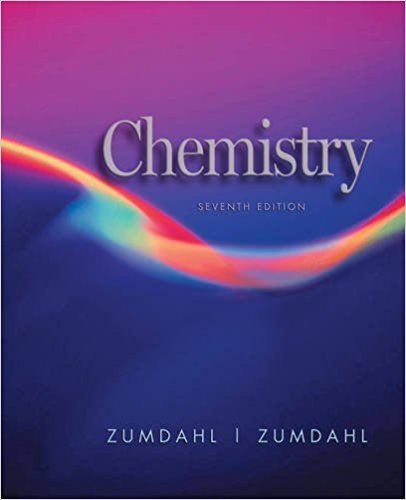×
×

# Solutions for Chapter 12: Chemical Kinetics## Full solutions for Chemistry | 7th Edition

ISBN: 9780618528448Solutions for Chapter 12: Chemical Kinetics

Solutions for Chapter 12
4 5 0 362 Reviews
23
5
##### ISBN: 9780618528448

Since 92 problems in chapter 12: Chemical Kinetics have been answered, more than 51369 students have viewed full step-by-step solutions from this chapter. Chapter 12: Chemical Kinetics includes 92 full step-by-step solutions. This expansive textbook survival guide covers the following chapters and their solutions. This textbook survival guide was created for the textbook: Chemistry, edition: 7. Chemistry was written by and is associated to the ISBN: 9780618528448.

Key Chemistry Terms and definitions covered in this textbook
• Aceto group

A CH3CO! group; also called an acetyl group

• alpha (a) helix

For proteins, a feature of secondary structure that forms when a portion of the protein twists into a spiral.

• Boyle’s law

A law stating that at constant temperature, the product of the volume and pressure of a given amount of gas is a constant. (Section 10.3)

In 13C NMR spectroscopy, a technique in which all 13C!1H splitting is suppressed with the use of two rf transmitters.

• chemical property.

Any property of a substance that cannot be studied without converting the substance into some other substance. (1.6)

• conjugate acid

A substance formed by addition of a proton to a Brønsted–Lowry base. (Section 16.2)

• Diol

A compound containing two hydroxyl groups

• Disproportionation

A termination process that involves the abstraction of a hydrogen atom from the beta position of the propagating radical of one chain by the radical endgroup of another chain.

In radical reaction mechanisms, a step in which a bond forms between the alpha (a) and beta (b) positions. As a result, a single bond at the b position is cleaved, causing the compound to fragment into two pieces.

• excited state

A state that is achieved when a compound absorbs energy.

• frontier orbital theory

The analysis of a reaction using MO theory, where only the frontier orbitals (HOMO and LUMO) are considered.

• gas constant (R)

The constant of proportionality in the ideal-gas equation. (Section 10.4)

• insulators

Materials that do not conduct electricity. (Section 12.7)

• intermolecular forces

The attractive forces between molecules.

• mass number

The sum of the number of protons and neutrons in the nucleus of a particular atom. (Section 2.3)

• Meisenheimer complex

Theresonance-stabilized intermediate of a nucleophilicaromatic substitution reaction.

• Pi (p) molecular orbital

A molecular orbital formed by overlapping parallel 2p orbitals on adjacent atoms; its electron density lies above and below the line connecting the atoms

• quartet

In NMR spectroscopy, a signal that is comprised of four peaks.

• specific rotation

For a chiral compound that is subjected to plane-polarized light, the observed rotation when a standard concentration (1 g/mL) and a standard path length (1 dm) are used.

• Valence Bond Theory

A model of bonding that places electron pairs between adjacent atoms to create bonds.

×